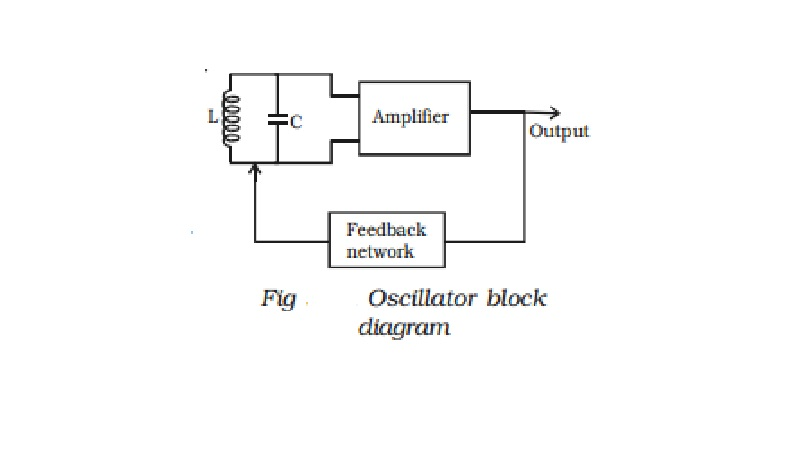Home | | Physics | | Physics | Essentials of LC oscillator

Essentials of LC oscillatorFig shows the block diagram of an oscillator. Its essential components are (i) tank circuit, (ii) amplifier and (iii) feedback circuit.

Essentials of LC oscillator

Fig  shows the block diagram of an oscillator. Its essential components are (i) tank circuit, (ii) amplifier and (iii) feedback circuit.

(i) Tank circuit : It consists of inductance coil (L) connected in parallel with capacitor (C). The

frequency of oscillations in the circuit depends upon the values of inductance coil and capacitance of the capacitor.

(ii) Amplifier : The transistor amplifier receives d.c. power from the battery and changes it into a.c. power for supplying to the tank circuit.

(iii) Feedback circuit : It provides positive feedback (i.e.) this circuit transfers a part of output energy to LC circuit in proper phase, to maintain the oscillations.LC oscillators

A transistor can work as an LC oscillator to produce undamped oscillations of any desired frequency, if tank and feedback circuits are properly connected to it. There are different LC oscillators used in electronic circuits, of which, the working principle of Colpitt's oscillator is discussed here.

Colpitt's oscillator

The circuit diagram of Colpitt's oscillator is shown in Fig. The resistance R1, R2 and RE provide the sufficient bias for the circuit The frequency determining network is the parallel resonant circuits consisting of capacitors C1, C2 and the inductor L. The junction of C1 and C2 is earthed. The function of the capacitor C4 is to block d.c and provide an a.c. path from the collector to the tank circuit. The voltage developed across C1 provides the positive feedback for sustained oscillations.

Study Material, Lecturing Notes, Assignment, Reference, Wiki description explanation, brief detail

Related Topics